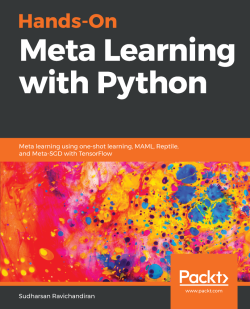# Hands-On Meta Learning with Python4.3 (3 reviews total)
By Sudharsan Ravichandiran
What do you get with a Packt Subscription?

• Constantly updated with 100+ new titles each month
• Breadth and depth in over 1,000+ technologies

Meta learning is an exciting research trend in machine learning, which enables a model to understand the learning process. Unlike other ML paradigms, with meta learning you can learn from small datasets faster.

Hands-On Meta Learning with Python starts by explaining the fundamentals of meta learning and helps you understand the concept of learning to learn. You will delve into various one-shot learning algorithms, like siamese, prototypical, relation and memory-augmented networks by implementing them in TensorFlow and Keras. As you make your way through the book, you will dive into state-of-the-art meta learning algorithms such as MAML, Reptile, and CAML. You will then explore how to learn quickly with Meta-SGD and discover how you can perform unsupervised learning using meta learning with CACTUs. In the concluding chapters, you will work through recent trends in meta learning such as adversarial meta learning, task agnostic meta learning, and meta imitation learning.

By the end of this book, you will be familiar with state-of-the-art meta learning algorithms and able to enable human-like cognition for your machine learning models.

Publication date:
December 2018
Publisher
Packt
Pages
226
ISBN
9781789534207

## Chapter 1. Introduction to Meta Learning

Meta learning is one of the most promising and trending research areas in the field of artificial intelligence right now. It is believed to be a stepping stone for attaining Artificial General Intelligence (AGI). In this chapter, we will learn about what meta learning is and why meta learning is the most exhilarating research in artificial intelligence right now. We will understand what is few-shot, one-shot, and zero-shot learning and how it is used in meta learning. We will also learn about different types of meta learning techniques. We will then explore the concept of learning to learn gradient descent by gradient descent where we understand how we can learn the gradient descent optimization using the meta learner. Going ahead, we will also learn about optimization as a model for few-shot learning where we will see how we can use meta learner as an optimization algorithm in the few-shot learning setting.

In this chapter, you will learn about the following:

• Meta learning
• Meta learning and few-shot
• Types of meta learning
• Optimization as a model for few-shot learning

## Meta learning

Meta learning is an exhilarating research domain in the field of AI right now. With plenty of research papers and advancements, meta learning is clearly making a major breakthrough in AI. Before getting into meta learning, let's see how our current AI model works.

Deep learning has progressed rapidly in recent years with great algorithms such as generative adversarial networks and capsule networks. But the problem with deep neural networks is that we need to have a large training set to train our model and it will fail abruptly when we have very few data points. Let's say we trained a deep learning model to perform task A. Now, when we have a new task, B, that is closely related to A, we can't use the same model. We need to train the model from scratch for task B. So, for each task, we need to train the model from scratch although they might be related.

Is deep learning really the true AI? Well, it is not. How do we humans learn? We generalize our learning to multiple concepts and learn from there. But current learning algorithms master only one task. Here is where meta learning comes in. Meta learning produces a versatile AI model that can learn to perform various tasks without having to train them from scratch. We train our meta learning model on various related tasks with few data points, so for a new related task, it can make use of the learning obtained from the previous tasks and we don't have to train them from scratch. Many researchers and scientists believe that meta learning can get us closer to achieving AGI. We will learn exactly how meta learning models learn the learning process in the upcoming sections.

### Meta learning and few-shot

Learning from fewer data points is called few-shot learning or k-shot learning where k denotes the number of data points in each of the classes in the dataset. Let's say we are performing the image classification of dogs and cats. If we have exactly one dog and one cat image then it is called one-shot learning, that is, we are learning from just one data point per class. If we have, say 10 images of a dog and 10 images of a cat, then that is called 10-shot learning. So k in k-shot learning implies a number of data points we have per class. There is also zero-shot learning where we don't have any data points per class. Wait. What? How can we learn when there are no data points at all? In this case, we will not have data points, but we will have meta information about each of the classes and we will learn from the meta information. Since we have two classes in our dataset, that is, dog and cat, we can call it two-way k-shot learning; so n-way means the number of classes we have in our dataset.

In order to make our model learn from a few data points, we will train them in the same way. So, when we have a dataset, D, we sample a few data points from each of the classes present in our data set and we call it as support set. Similarly, we sample some different data points from each of the classes and call it as query set. So we train our model with a support set and test with a query set. We train our model in an episodic fashion—that is, in each episode, we sample a few data points from our dataset, D, prepare our support set and query set, and train on the support set and test on the query set. So, over series of episodes, our model will learn how to learn from a smaller dataset. We will explore this in more detail in the upcoming chapters.

## Types of meta learning

Meta learning can be categorized in several ways, right from finding the optimal sets of weights to learning the optimizer. We will categorize meta learning into the following three categories:

• Learning the metric space
• Learning the initializations
• Learning the optimizer

### Learning the metric space

In the metric-based meta learning setting, we will learn the appropriate metric space. Let's say we want to learn the similarity between two images. In the metric-based setting, we use a simple neural network that extracts the features from two images and finds the similarity by computing the distance between features of these two images. This approach is widely used in a few-shot learning setting where we don't have many data points. In the upcoming chapters, we will learn about metric-based learning algorithms such as Siamese networks, prototypical networks, and relation networks.

### Learning the initializations

In this method, we try to learn optimal initial parameter values. What do we mean by that? Let's say we are a building a neural network to classify images. First, we initialize random weights, calculate loss, and minimize the loss through a gradient descent. So, we will find the optimal weights through gradient descent and minimize the loss. Instead of initializing the weights randomly, if can we initialize the weights with optimal values or close to optimal values, then we can attain the convergence faster and we can learn very quickly. We will see how exactly we can find these optimal initial weights with algorithms such as MAML, Reptile, and Meta-SGD in the upcoming chapters.

### Learning the optimizer

In this method, we try to learn the optimizer. How do we generally optimize our neural network? We optimize our neural network by training on a large dataset and minimize the loss using gradient descent. But in the few-shot learning setting, gradient descent fails as we will have a smaller dataset. So, in this case, we will learn the optimizer itself. We will have two networks: a base network that actually tries to learn and a meta network that optimizes the base network. We will explore how exactly this works in the upcoming sections.

Now, we will see one of the interesting meta learning algorithms called learning to learn gradient descent by gradient descent. Isn't the name kind of daunting? Well, in fact, it is one of the simplest meta learning algorithms. We know that, in meta learning, our goal is to learn the learning process. In general, how do we train our neural networks? We train our network by computing loss and minimizing the loss through gradient descent. So, we optimize our model using gradient descent. Instead of using gradient descent can we learn this optimization process automatically?

But how can we learn this? We replace our traditional optimizer (gradient descent) with the Recurrent Neural Network (RNN). But how does this work? How can we replace gradient descent with RNN? If you examine closely, what are we really doing in gradient descent? It is basically a sequence of updates from the output layer to the input layer and we store these updates in a state. So, we can use RNN and store the updates in an RNN cell.

So, the main idea of this algorithm is to replace gradient descent with RNN. But the question is how do RNNs learn? How can we optimize the RNN? For optimizing an RNN, we use gradient descent. So, in a nutshell, we are learning to perform gradient descent through an RNN and that RNN is optimized by gradient descent and that's what is meant by the name learning to learn gradient descent by gradient descent.

We call our RNN, an optimizer and our base network, an optimizee. Let's say we have a modelparameterized by some parameter. We need to find this optimal parameter, so that we can minimize the loss. In general, we find this optimal parameter through gradient descent, but now we use the RNN for finding this optimal parameter. So the RNN (optimizer) finds the optimal parameter and sends it to the optimizee (base network); the optimizee uses this parameter, computes the loss, and sends the loss to the RNN. Based on the loss, the RNN optimizes itself through gradient descent and updates the model parameter.

Confusing? Look at the following diagram: our optimizee (base network) is optimized through our optimizer (RNN). The optimizer sends the updated parameters—that is, weights—to the optimizee and the optimizee uses these weights, calculates the loss, and sends the loss to the optimizer; based on the loss, the optimizer improves itself through gradient descent: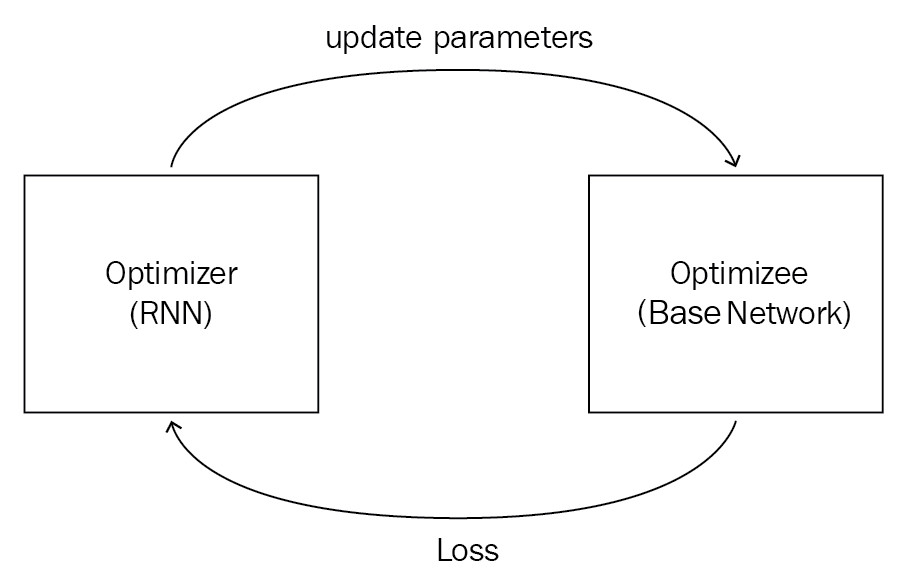Let's say our base network (optimizee) is parameterized byand our RNN (optimizer) is parameterized by. What is the loss function of the optimizer? We know that the optimizer's role (RNN) is to reduce the loss of the optimizee (base network). So the loss of our optimizer is the average loss of the optimizee and it can be represented as follows:How do we minimize this loss? We minimize this loss through gradient descent by finding the right. Okay, what does the RNN take as input and what output would it return? Our optimizer, that is, our RNN, takes as input the gradient of optimizeeas well as its previous stateand returns output, an updatethat can minimize the loss of our optimizee. Let's denote our RNN by a function:In the previous equation, the following applies:

•is the gradient of our model (optimizee), that is,•is the hidden state of the RNN
•is the parameter for the RNN
• Outputsandis the update and next state of the RNN respectively

So, we update our model parameter values using.

As you can see in the following diagram, our optimizerat a time t, takes in a hidden stateasas inputs, computesand sends it to our optimizee, where it is added withand becomesfor an update at the next time step: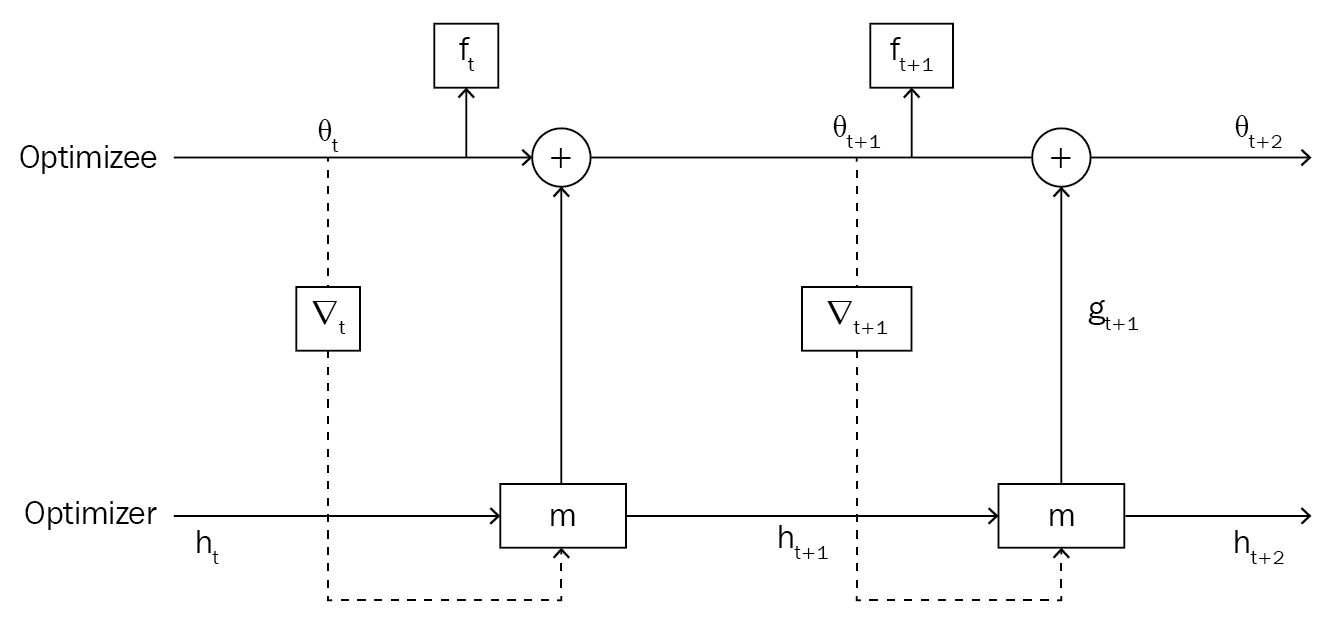So, in this way, we learn the gradient descent optimization through gradient descent.

## Optimization as a model for few-shot learning

We know that, in few-shot learning, we learn from lesser data points, but how can we apply gradient descent in a few-shot learning setting? In a few-shot learning setting, gradient descent fails abruptly due to very few data points. Gradient descent optimization requires more data points to reach the convergence and minimize loss. So, we need a better optimization technique in the few-shot regime. Let's say we have amodel parameterized by some parameter. We initialize this parameterwith some random values and try to find the optimal value using gradient descent. Let's recall the update equation of our gradient descent:In the previous equation, the following applies:

•is the updated parameter
•is the parameter value at previous time step
•is the learning rate
•is the gradient of loss function with respect toDoesn't the update equation of gradient descent look familiar? Yes, you guessed it right: it resembles the cell state update equation of LSTM and it can be written as follows:We can totally relate our LSTM cell update equation with gradient descent as, let's say= 1, then the following applies:So, instead of using gradient descent as an optimizer in the few-shot learning regime, we can use LSTM as an optimizer. Our meta learner is the LSTM, which learns the update rule for training our model. So we use two networks: one, our base learner, which learns to perform a task, and the other, the meta learner, which tries to find the optimal parameter. But how does this work?

We know that, in LSTM, we use a forget gate for discarding information that is not required in the memory, and it can be represented as follows:How can this forget gate be useful in our optimization setting? Let's say we are in a position where the loss is high, and the gradient is close to zero. How can we escape from this position? In this case, we can shrink the parameters of our model and forget some parts of its previous value. So, we can use our forget gate to do that and it takes a current parameter value, current lossand the previous forget gate as the input; it can be represented as follows:Now let's come to the input gate. We know that the input gate in LSTM is used for deciding what value to update, and it can be represented as follows:In our few-shot learning setting, we can use this input gate to tune our learning rate to learn quickly while preventing it from divergence:So, our meta learner learns the optimal value ofandBut still, how does this work?

Let's say we have a base networkparameterized byand our LSTM meta learnerparameterized by. Assume that we have a dataset. We split our dataset asandfor training and testing respectively. First, we randomly initialize our meta learner parameter.

For some T number of iterations, we randomly sample data points from, calculate the loss, and then we calculate the gradients of loss with respect to our model parameter. Now we feed this gradient, loss, and meta learner parameterto our meta learner. Our meta learnerwill return a cell stateand then we update our base networkparameterat a time t as.We repeat this for some N number of times, as shown in the following diagram: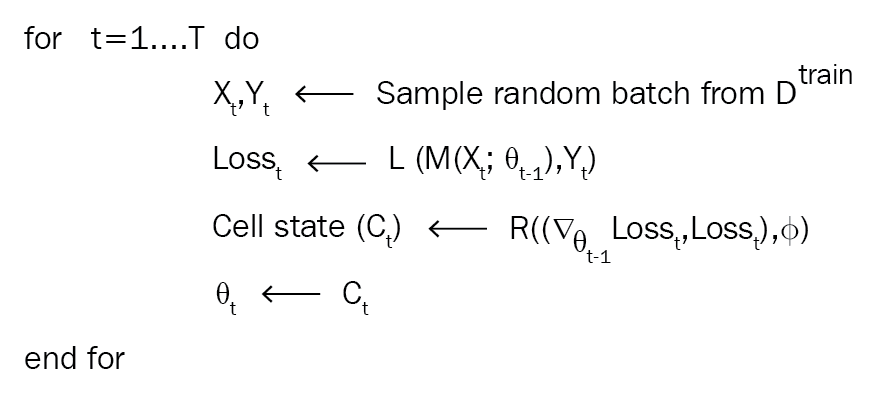So, after T iterations, we will have an optimal parameter. But how can we check the performance ofand how can we update our meta learner parameter? We take the test set and compute the loss on our test set with parameter. Then, we calculate the gradients of the loss with respect to our meta learner parameterand then we update, as shown here: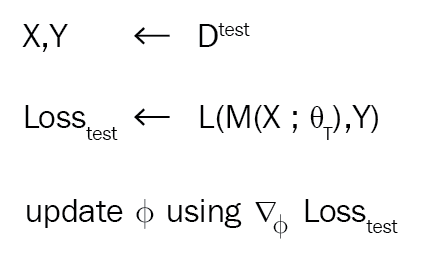We do this for some n number of iterations and update our meta learner. The overall algorithm is shown here: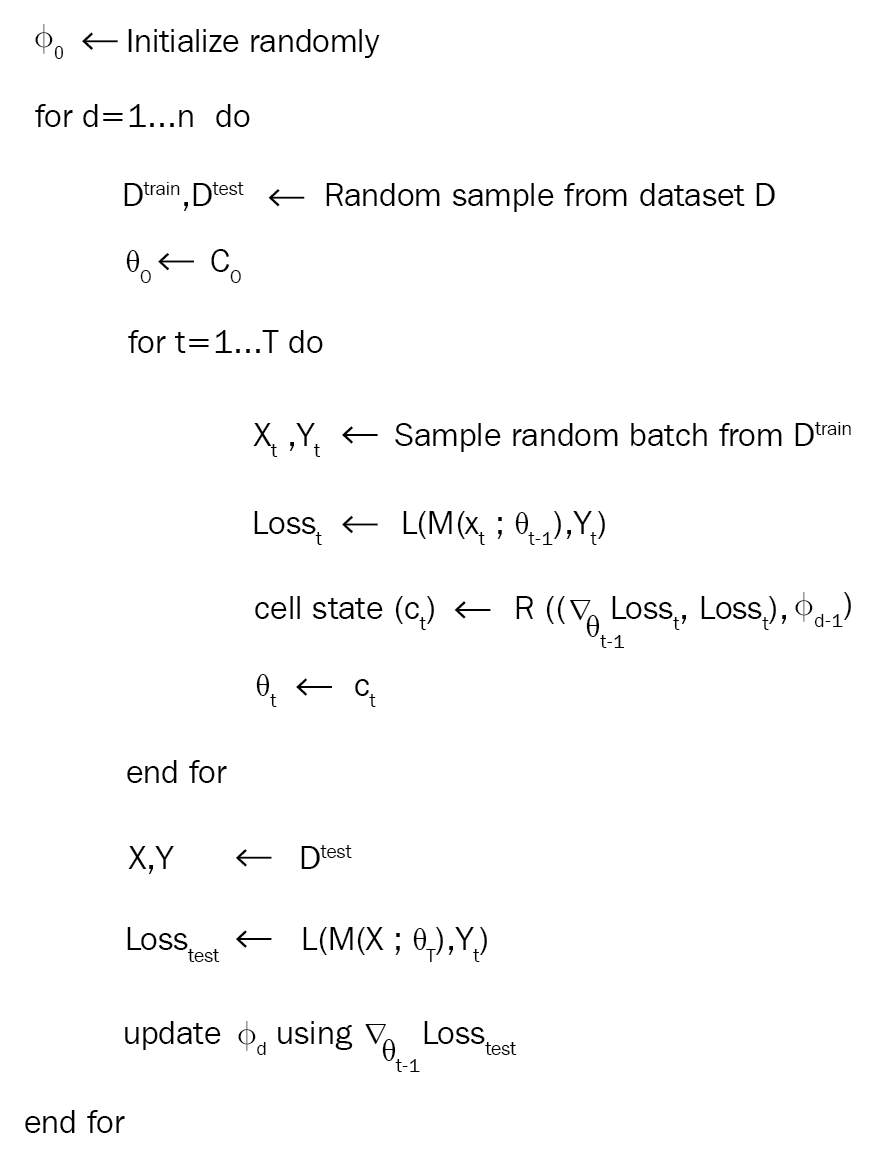## Summary

We started off by understanding what meta learning is and how one-shot, few-shot, and zero-shot learning is used in meta learning. We learned that the support set and query set are more like a train set and test set but with k data points in each of the classes. We also saw what n-way k-shot means. Later, we understood different types of meta learning techniques. Then, we explored learning to learn gradient descent by gradient descent where we saw how RNN is used as an optimizer to optimize the base network. Later, we saw optimization as a model for few-shot learning where we used LSTM as a meta learner for optimizing in the few-shot learning setting.

In the next chapter, we will learn about a metric-based meta learning algorithm called the Siamese network and we will see how to use a Siamese network for performing face and audio recognition.

## Questions

1. What is meta learning?
2. What is few-shot learning?
3. What is a support set?
4. What is a query set?
5. What is metric-based learning called?
6. How do we perform training in meta learning?